Finite Geometry Notes   | Home | Site Map | Author |

Keys for search: "triangle tiling," "n2 congruent triangles [i.e., n 2 ]," Cullinane, Soifer, Beeson

Triangles Are Square

by Steven H. Cullinane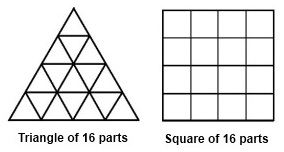A triangle-decomposition result from 1984:

 American Mathematical Monthly, June-July 1984, p. 382 MISCELLANEA, 129 Triangles are square "Every triangle consists of  n congruent copies of itself" is true if and only if  n is a square. (The proof is trivial.) -- Steven H. Cullinane

A followup from 1985:

 American Mathematical Monthly, Vol. 92, No. 6 (June-July 1985), p. 443 LETTERS TO THE EDITOR Material  for this department should be prepared exactly the same way as submitted manuscripts (see the inside front cover) and sent to Professor P. R. Halmos, Department of Mathematics, University of Santa Clara, Santa Clara, CA 95053 Editor:     Miscellaneum 129 ("Triangles are square," June-July 1984 Monthly ) may have misled many readers. Here is some background on the item.     That n2 points fall naturally into a triangular array is a not-quite-obvious fact which may have applications (e.g., to symmetries of Latin-square "k-nets") and seems worth stating more formally. To this end, call a convex polytope P  an n-replica  if  P  consists of n mutually congruent polytopes similar to P  packed together. Thus, for n ∈ ℕ,     (A) An equilateral triangle is an n-replica if and only if n is a square.     Does this generalize to tetrahedra, or to other triangles? A regular tetrahedron is not a (23)-replica, but a tetrahedron ABCD  with edges AB, BC, and CD  equal and mutually orthogonal is an n-replica if and only if n is a cube. Every triangle satisfies the "if" in (A), so, letting T  be the set of triangles, one might surmise that     (B) ∀ t ∈ T (t is an n-replica if and only if n is a square).      This, however, is false. A. J. Schwenk has pointed out that for any m ∈ ℕ, the 30°-60°-90° triangle is a (3m2)-replica, and that a right triangle with legs of integer lengths a and b is an ((a2 + b2)m2)-replica. As Schwenk notes, it does not seem obvious which other values of n can occur in counterexamples to (B). Shifting parentheses to fix (B), we get a "square-triangle" lemma:     (C) (∀ t ∈ T, t  is an n-replica) if and only if n is a square.         Miscellaneum 129 was a less formal statement of (C), with quotation marks instead of parentheses; this may have led many readers to think (B) was intended. To these readers, my apology. Steven H. Cullinane       501 Follett Run Road      Warren, PA 16365

The above 1985 note immediately suggests a problem—

What mappings of a square array of n 2 points to
a triangular array of n 2 points are "natural"?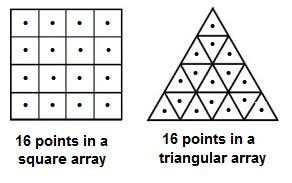(In the figure above, whether the 322,560 natural permutations
of the 16 points in the square map in any natural way
to permutations of the 16 points in the triangle
is not immediately apparent.)

Here is a trial solution of the inverse problem—Another trial mapping, from square to triangle,
based on gnomons of the square: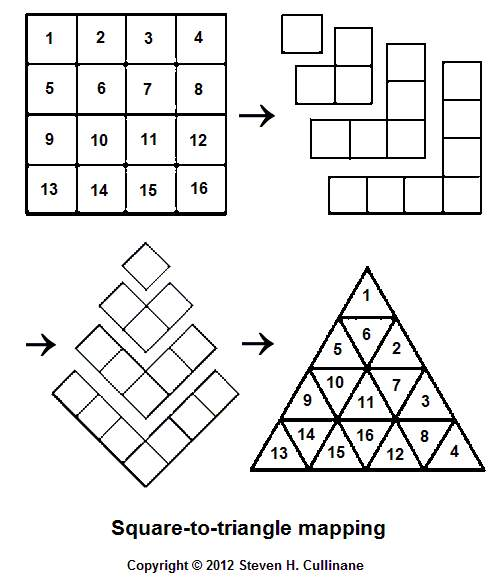Problem— Devise a test for "naturality" of such mappings
and apply it to the trial mappings above.

Update of July 16, 2012:

Another approach to the
square-to-triangle mapping problem —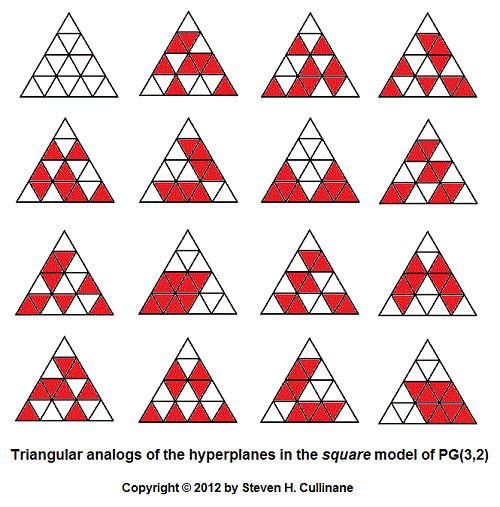For the square model referred to in the above picture, see (for instance)

Coordinates for the 16 points in the triangular arrays
of the corresponding affine space may be deduced from
the patterns in the projective-hyperplanes array above.

This solves the inverse problem of mapping,
in a natural way, the triangular array of 16 points
to the square array of 16 points.

Note that the square model's 15 hyperplanes S
and the triangular model's 15 hyperplanes T —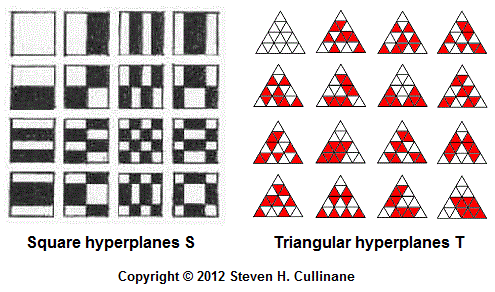— share the following vector-space structure —

 0 c d c + d a a + c a + d a + c + d b b + c b + d b + c + d a + b a + b + c a + b + d a + b +    c + d

(This vector-space a b c d  diagram is from
Chapter 11 of   Sphere Packings, Lattices
and Groups
, by   John Horton Conway and
in 1988.)

Update of March 26, 2012:

For related material, see

Beeson,  Michael, Triangle Tiling , series of preprints, 2012

Soifer, Alexander, How Does One Cut a Triangle?, second edition, Springer, 2009

As of March 26, 2012, neither Soifer nor Beeson have referenced the 1985 Monthly letter.

Page created January 16, 2012.